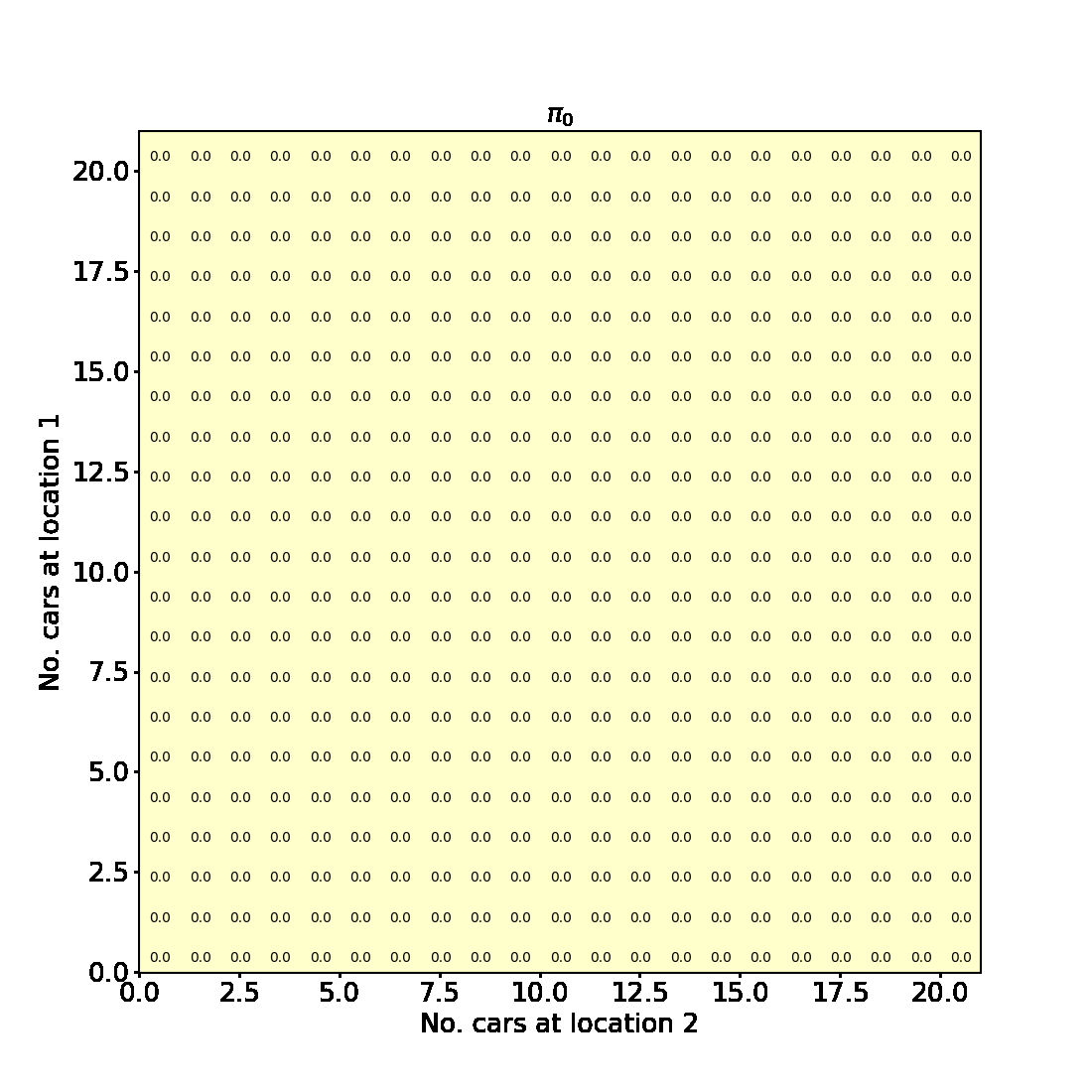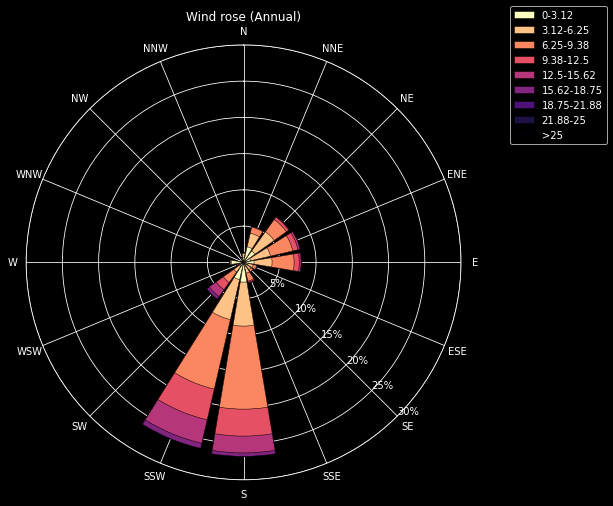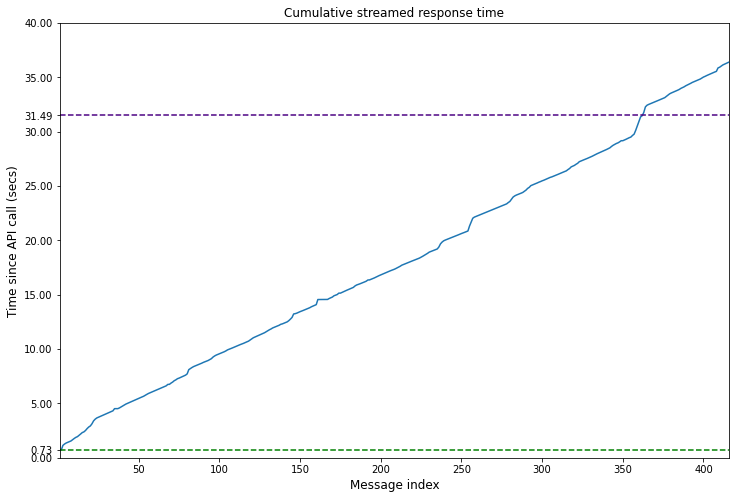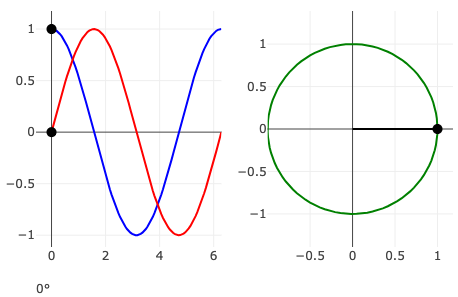### A Minimalist ChatGPT for Jupyter Notebook or Command Line

In this blog post, we will explore how to implement a minimalist ChatGPT-style app in a Jupyter Notebook. The goal is to provide an understanding of the important concepts, components, and techniques required to create a chat app on top of a large language model (LLM), specifically OpenAI’s GPT.### Policy Iteration for finite MDPs

In this blogpost we will implement Example 4.2 from Chapter 4 Reinforcement Learning (Sutton and Barto aka the RL book). This is an example of a problem involving a finite Markov Decision Process for which policy iteration is used to find an optimal policy.### How to use curly brackets {} in LaTeX

In this tutorial, we will discuss various ways to use curly brackets $\{\}$ in LaTeX. The simplest case involves using curly brackets to denote a set. However, curly brackets, or braces, can also be used to group multiple lines of calculations and mathematical equations or to add explanatory text above or below the expressions.### How to plot a Windrose in Python

A windrose is a circular histogram that shows the distribution of wind speeds and directions over a period of time. In this tutorial we will learn how to plot a windrose in Python using Matplotlib and Plotly.### How to stream ChatGPT responses from the OpenAI API

In this blog post we will learn how you can replicate this experience of the ChatGPT web application by streaming responses from the OpenAI API.### Animations in Plotly.js

In this blog, we will demystify the process of creating interactive animations using Plotly.js.### Writing Sums, Products and Integrals in LaTeX

In this blog post, we will learn how to write sums, products, and various types of integrals using LaTeX.### Representing Norms in LaTeX

In this blog post, we will learn how to write the norm function in LaTeX and how to represent different types of norms.### Writing Ceil, Floor and Abs in LaTeX

In this blog post, we will learn how to write the ceiling, floor and absolute value functions in LaTeX, both in mathematical notation and as text.In this blog post, we’ll explore how to define convenient shortcuts using \newcommand in LaTeX, making your notation more concise and readable.